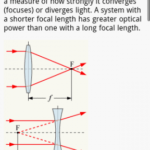# How to study Physics better, Move towards ConceptsWe are quite accustomed to reading history, geography, English easily but it is usually the science subjects which leave us hopeless. And most difficult of them is physics which goes over our head.But it not like that. Physics is most interesting subject and we ought to study it as everything in this universe is governed by it. It only requires a scientific approach with a mathematical bent of mind. Here is how it is possible.

• Students usually get bogged up with the size of the books and the lengthy formulas. But study of physics is not required in a single shot. In fact, the book is usually broken into chunks and pieces of information which is aggregated into one place. Thus, one topic is to be read at once.
• There are plenty of physics books in the market, and everyone gives a new suggestion regarding a particular book being the best. But only that book should be chosen which we understand properly and easily. Choosing the right book is a basic requirement.
• Before a study of particular chapter is started, a small glance through the whole chapter should be made which would make us familiar with it. It would give the basic idea.
• Then the study should be started with a pen and a pad in a hand. Every bit of information read should be noted down in the form of points in chronology. This would reduce the whole matter into mere sentences.
• Before reading any derivation, the concept should be well understood, and the significance of each and every symbol should be known. Then the step by step derivation should be read and once it is convinced that the derivation is properly understood, it should be performed in copy by own self.
• For numerical, two things are required, first is understanding the concept and second is remembering the formulas. Concepts can be understood, but it is usually remembering the formula where problems are faced. So large charts containing all important formulas should be made and pasted on the wall which should be glanced every day. This process would make us remember formulas easily.
• As we all tend to retain information more easily when we visualize it. So every concept should be practiced as a diagram such as free body diagram, ray diagram, energy diagram.
• Along with the academic study we must also indulge in practical science. Performing small experiments will make us understand the concept better.
• The solved examples should be read very properly and should be practiced by own self in the notebook.
• Then, we must find the similarities between the concepts to retain better. For example, coulomb’s law and Newton’s law in gravitation are different but the basics behind them are very similar. So understanding one topic easily will help in the other topic.
• Having a good guide is very important. The guide must understand our requirements and must guide us accordingly to whom we can turn to during problems.

Now, studying physics is made easier. So we should keep on studying and keep on exploring.

Topic:  Other